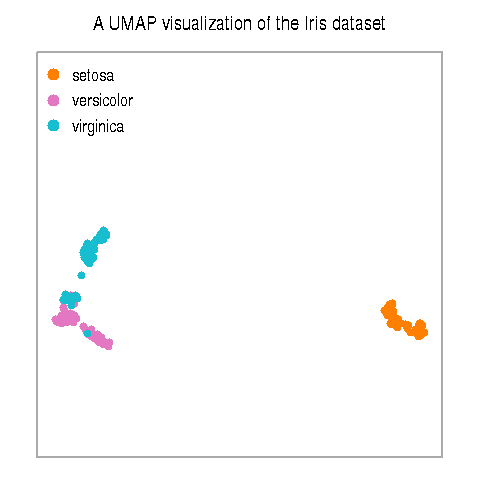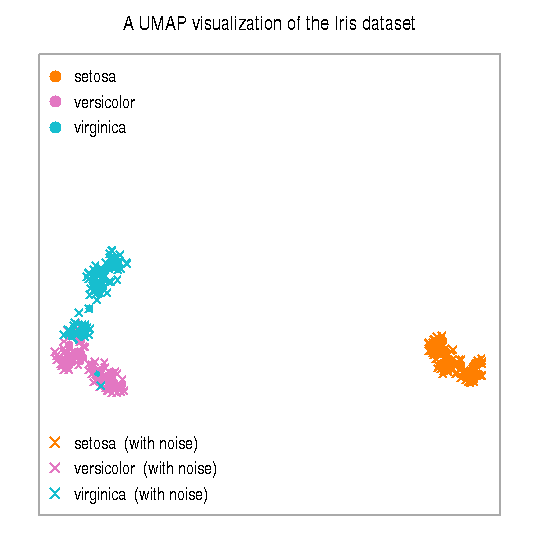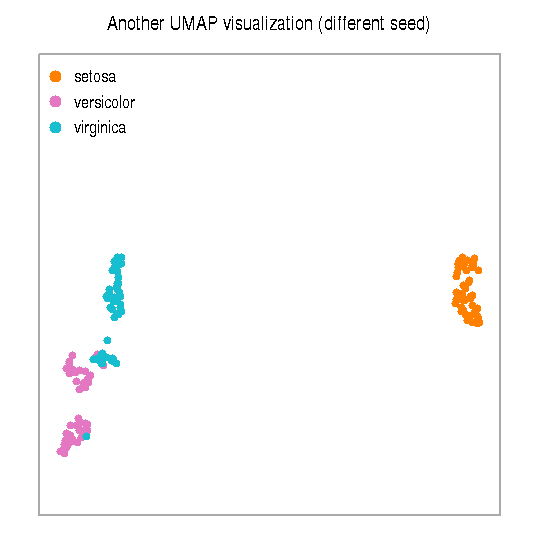# Uniform Manifold Approximation and Projection in R

## Introduction

Uniform Manifold Approximation and Projection (UMAP) is an algorithm for dimensional reduction. Its details are described by McInnes, Healy, and Melville and its official implementation is available through a python package umap-learn. The R package `umap` described in this vignette is a separate work that provides two implementations for using UMAP within the R environment. One implementation is written from-scratch and another links to the official umap-learn. (Another R package, uwot, provides a separate implementation with a slightly different interface).

The vignette covers basic usage, tuning, stability and reproducibility, and discusses toggling between different implementations. Throughout, the vignette uses a small dataset as an example, but the package is suited to processing larger data with many thousands of data points.

## Overview

For a practical demonstration, let’s use the Iris dataset.

``head(iris, 3)``
``````##   Sepal.Length Sepal.Width Petal.Length Petal.Width Species
## 1          5.1         3.5          1.4         0.2  setosa
## 2          4.9         3.0          1.4         0.2  setosa
## 3          4.7         3.2          1.3         0.2  setosa``````

The first four columns contain data and the last column contains a label. It will be useful to separate those components.

``````iris.data = iris[, grep("Sepal|Petal", colnames(iris))]
iris.labels = iris[, "Species"]``````

### Creating a projection

Let’s load the `umap` package and apply the UMAP transformation.

``````library(umap)
iris.umap = umap(iris.data)``````

The output is an object and we can get a summary of its contents by printing it.

``iris.umap``
``````## umap embedding of 150 items in 2 dimensions
## object components: layout, data, knn, config``````

The main component of the object is ‘layout’, which holds a matrix with coordinates.

``head(iris.umap\$layout, 3)``
``````##          [,1]      [,2]
## [1,] 15.95804 -1.418806
## [2,] 17.80407 -1.531492
## [3,] 17.59992 -2.239721``````

These coordinates can be used to visualize the dataset. (The custom plot function, `plot.iris`, is available at the end of this vignette.)

``plot.iris(iris.umap, iris.labels)``### Projecting new data

Once we have a ‘umap’ object describing an embedding of a dataset into a low-dimensional layout, we can project other data onto the same manifold.

To demonstrate this, we need a second dataset with the same data features as the training data. Let’s create such data by adding some noise to the original.

``````iris.wnoise = iris.data + matrix(rnorm(150*40, 0, 0.1), ncol=4)
colnames(iris.wnoise) = colnames(iris.data)
``````##   Sepal.Length Sepal.Width Petal.Length Petal.Width
## 1     5.183373    3.525821     1.515123   0.3285499
## 2     4.872395    3.096451     1.467537   0.2094982
## 3     4.664500    3.150872     1.421417   0.2190057``````

We can now arrange these perturbed observations onto the same layout as before. Following R’s design pattern for fitted models, this is performed via `predict`.

``````iris.wnoise.umap = predict(iris.umap, iris.wnoise)
``````##       [,1]      [,2]
## 1 15.30520 -1.921424
## 2 18.09914 -1.361412
## 3 17.47446 -2.782222``````

The output is a matrix with coordinates. We can visualize these points alongside the original.

``````plot.iris(iris.umap, iris.labels)
plot.iris(iris.wnoise.umap, iris.labels, add=T, pch=4, legend.suffix=" (with noise)")``````The new observations lie close to their original counterparts.

## Tuning

The example above uses function `umap` with a single argument - the input dataset - so the embedding is performed with default settings. However, the algorithm can be tuned via configuration objects or via additional arguments.

### Configuration objects

The default configuration object is called `umap.defaults`. This is a list encoding default values for all the parameters used by the algorithm.

``umap.defaults``
``````## umap configuration parameters
##            n_neighbors: 15
##           n_components: 2
##                 metric: euclidean
##               n_epochs: 200
##                  input: data
##                   init: spectral
##               min_dist: 0.1
##       set_op_mix_ratio: 1
##     local_connectivity: 1
##              bandwidth: 1
##                  alpha: 1
##                  gamma: 1
##   negative_sample_rate: 5
##                      a: NA
##                      b: NA
##           random_state: NA
##        transform_state: NA
##                    knn: NA
##            knn_repeats: 1
##                verbose: FALSE
##        umap_learn_args: NA``````

For a description of each field, see the documentation in `help(umap.defaults)` or the original publication.

To create a custom configuration, we can make a copy of the default object and then update its fields. For example, let’s change the seed for random number generation.

``````custom.config = umap.defaults
custom.config\$random_state = 123``````

We can observe the changed settings by inspecting the object again (try it). To perform the UMAP projection with these settings, we can run the projection again and provide the configuration object as a second argument.

``````iris.umap.config = umap(iris.data, config=custom.config)
plot.iris(iris.umap.config, iris.labels,
main="Another UMAP visualization (different seed)")``````The result is slightly different than before due to a new instantiation of the random number generator.

Another way to customize the algorithm is to specify the parameter values one-by-one as arguments to the function call. The command below achieves equivalent results to the above.

``iris.umap.args = umap(iris.data, random_state=123)``

The coordinates in this output object should match the ones from `iris.umap.config` (check it!).

Behind the scenes, the `umap` function call extracts most of the parameter values from the default configuration, and then replaces the value of `random_state` with the newly specified value. It is also possible to use a custom configuration and parameter values together.

### Input types

All the examples above apply the umap transformation on a raw dataset. The first stage in the algorithm is the calculation of nearest neighbors and distances between them. In cases when these distances or nearest neighbors are known a-priori, the `umap` function can start with those inputs instead.

To use a distance matrix instead of raw data, we can set the `input` argument to ‘dist’.

``````iris.dist = as.matrix(dist(iris.data))
iris.umap.dist = umap(iris.dist, config=custom.config, input="dist")
iris.umap.dist``````
``````## umap embedding of 150 items in 2 dimensions
## object components: layout, data, knn, config``````

Because `iris.umap.dist` is computed with the custom configuration, it holds the same embedding layout as `iris.umap.config` above (check it!). However, this object does not carry the original data. As such, it cannot be used to ‘predict’ new data into the embedding: attempting a command like `predict(iris.umap.dist, iris.wnoise)` will generate an error. This is because the algorithm will not have sufficient information to computing distances between new data points and previously embedded data.

Another way to provide data to function `umap` is via precomputed nearest neighbors. As we saw above, each object of class ‘umap’ has a component called ‘knn’. We can preview this component by printing it.

``iris.umap\$knn``
``````## k-nearest neighbors for 150 items with k=15
## object components: indexes, distances
## Note: nearest neighbors may be approximate``````

This is actually a list with two matrices. One matrix associates each data point to a fixed number of nearest neighbors (the default is 15). The other matrix specifies the distances between them.

To see how to use this data structure, let’s construct an object describing 10 nearest neighbors, and then perform an embedding.

``````# extract information on 10 nearest neighbors from iris.umap
iris.neighbors = iris.umap\$knn\$indexes[, 1:10]
iris.neighbors.distances = iris.umap\$knn\$distances[, 1:10]
# construct an object with indexes and distances
iris.knn.10 = umap.knn(indexes=iris.neighbors, distances=iris.neighbors.distances)
iris.knn.10``````
``````## k-nearest neighbors for 150 items with k=10
## object components: indexes, distances
## Note: nearest neighbors may be approximate``````
``````# perform an embedding using the precomputed nearest neighbors
iris.umap.knn = umap(iris.data, config=custom.config, n_neighbors=10, knn=iris.knn.10)``````

In this function call, argument `config` specifies most of the parameter values. Because the custom configuration has `n_neighbors` at 15 and here we want to use 10 instead, the next argument sets this new value. The final argument provides our prepared object. Functions `umap` detect the availability of this object and can skip calculating nearest neighbors. This can substantially improve running times.

## Stability and reproducibility

The UMAP algorithm uses random numbers and thus results may vary from run to run. As we have seen, it is possible to obtain a minimal level of reproducibility by setting seeds. Nonetheless, there are some subtle points worth covering in more detail.

### Initial embedding

As shown in the section on tuning, embedding of a raw dataset can be stabilized by setting a seed for random number generation. However, the results are stable only when the raw data are re-processed in exactly the same way. Results are bound to change if data are presented in a different order.

A separate consideration is about the effect of the randomness within the umap algorithms on the state of the random-number generator in the calling environment. By default, the `umap` function preserves the external random state. This has some pros and cons in various situations. One important consequence of the default setting is that when two calls are made `umap` one after the other, they both receive the same random state. If this is not a desired behavior, those calls should be made either with their own explicit seeds, or setting argument `preserve.seed=FALSE`.

### Prediction

Projection of new data onto an existing embedding uses a separate random number generator, which is controlled via parameter `transform_state`. This seed ensures that predictions executed in exactly the same way produce identical output each time. The default algorithm further implements a mechanism by which predictions are consistent when performed individually or in batch. Let’s look at an example based on the Iris data.

``````# predict in batch, display first item
predict(iris.umap, iris.wnoise)[1, , drop=FALSE]``````
``````##      [,1]      [,2]
## 1 15.3052 -1.921424``````
``````# predict only first item
predict(iris.umap, iris.wnoise[1,,drop=FALSE])``````
``````##      [,1]      [,2]
## 1 15.3052 -1.921424``````

The mechanism that enables stability between batch and individual predictions also ensures that predictions are stable when data are presented in a different order. However, this mechanism is not enforced (for performance reasons) when the new data contain more than a thousand rows. This is an implementation detail and may change in future versions.

## Implementations

The package provides two implementations of the umap method, one written in R (with help from several packages from CRAN) and one accessed via an external python module.

The implementation written in R is the default. It follows the design principles of the UMAP algorithm and its running time scales better-than-quadratically with the number of items (points) in a dataset. It is thus in principle suitable for use on datasets with thousands of points. This implementation is the default because it should be functional without extensive dependencies.

The second available implementation is a wrapper for a python package `umap-learn`. To enable this implementation, specify the argument `method`.

``iris.umap.learn = umap(iris.data, method="umap-learn")``

This command has several dependencies. The `reticulate` package must be installed and loaded (use `install.packages("reticulate")` and `library(reticulate)`). Furthermore, the `umap-learn` python package must be available (see the package repository for instructions). If either of these components is not available, the above command will display an error message.

A separate vignette explains additional aspects of interfacing with `umap-learn`, including handling of discrepancies between releases.

Note that it will not be possible to produce exactly the same output from the two implementations due to inequivalent random number generators in R and python, and due to slight discrepancies in the implementations.

## Appendix

The custom plot function used to visualize the Iris dataset:

``plot.iris``
``````## function(x, labels,
##          main="A UMAP visualization of the Iris dataset",
##          colors=c("#ff7f00", "#e377c2", "#17becf"),
##          cex.main=1, cex.legend=0.85) {
##
##   layout = x
##   if (is(x, "umap")) {
##     layout = x\$layout
##   }
##
##   xylim = range(layout)
##   xylim = xylim + ((xylim-xylim)*pad)*c(-0.5, 0.5)
##     par(mar=c(0.2,0.7,1.2,0.7), ps=10)
##     plot(xylim, xylim, type="n", axes=F, frame=F)
##     rect(xylim, xylim, xylim, xylim, border="#aaaaaa", lwd=0.25)
##   }
##   points(layout[,1], layout[,2], col=colors[as.integer(labels)],
##          cex=cex, pch=pch)
##   mtext(side=3, main, cex=cex.main)
##
##   labels.u = unique(labels)
##   legend.pos = "topleft"
##   legend.text = as.character(labels.u)
##     legend.pos = "bottomleft"
##     legend.text = paste(as.character(labels.u), legend.suffix)
##   }
##
##   legend(legend.pos, legend=legend.text, inset=0.03,
##          col=colors[as.integer(labels.u)],
##          bty="n", pch=pch, cex=cex.legend)
## }
## <bytecode: 0x55bca5af9d58>``````

Summary of R session:

``sessionInfo()``
``````## R version 3.6.1 (2019-07-05)
## Platform: x86_64-pc-linux-gnu (64-bit)
## Running under: Ubuntu 18.04.5 LTS
##
## Matrix products: default
## BLAS:   /software/opt/R/R-3.6.1/lib/libRblas.so
## LAPACK: /software/opt/R/R-3.6.1/lib/libRlapack.so
##
## locale:
##   LC_CTYPE=en_GB.UTF-8       LC_NUMERIC=C
##   LC_TIME=en_GB.UTF-8        LC_COLLATE=C
##   LC_MONETARY=en_GB.UTF-8    LC_MESSAGES=en_GB.UTF-8
##   LC_PAPER=en_GB.UTF-8       LC_NAME=C
##  LC_MEASUREMENT=en_GB.UTF-8 LC_IDENTIFICATION=C
##
## attached base packages:
##  stats     graphics  grDevices utils     datasets  methods   base
##
## other attached packages:
##  umap_0.2.7.0
##
## loaded via a namespace (and not attached):
##   Rcpp_1.0.5      lattice_0.20-38 digest_0.6.23   RSpectra_0.16-0
##   grid_3.6.1      jsonlite_1.7.1  magrittr_1.5    evaluate_0.14
##   rlang_0.4.4     stringi_1.4.3   Matrix_1.2-18   reticulate_1.18
##  rmarkdown_2.0   tools_3.6.1     stringr_1.4.0   xfun_0.11
##  yaml_2.2.0      compiler_3.6.1  askpass_1.1     htmltools_0.5.0
##  openssl_1.4.3   knitr_1.26``````